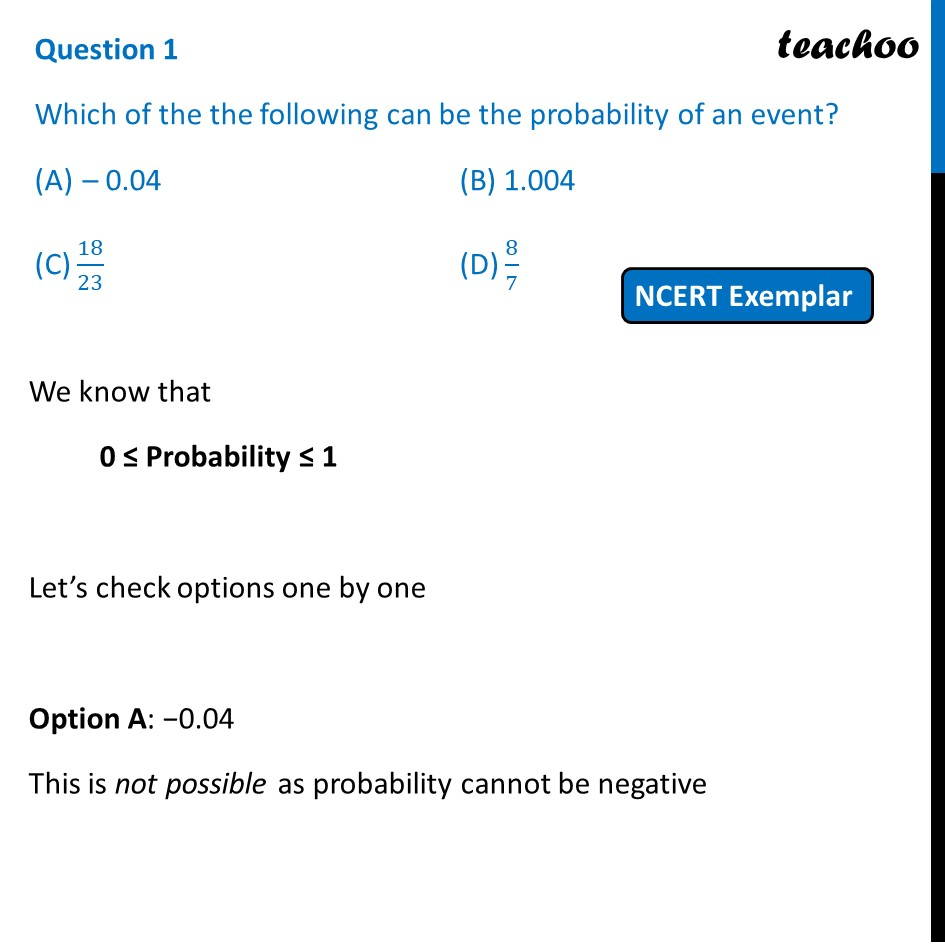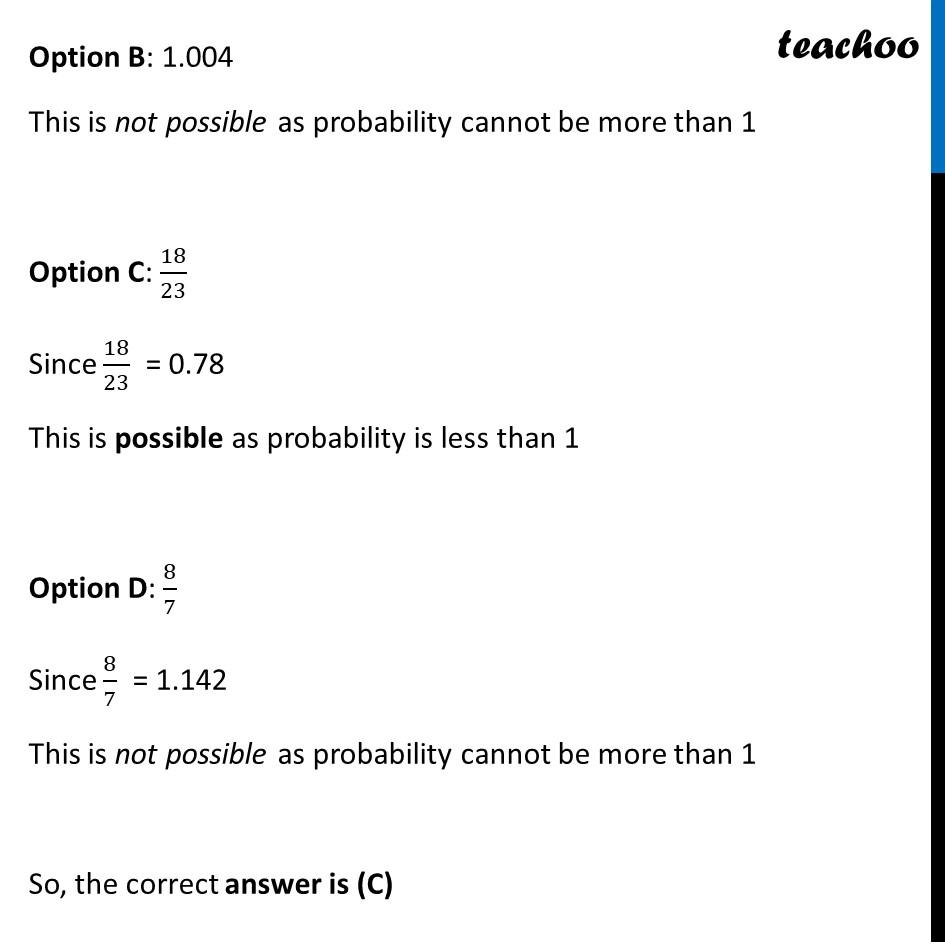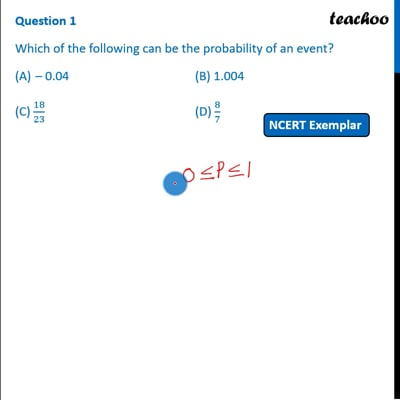NCERT Exemplar - MCQ

Chapter 15 Class 10 Probability
Serial order wise

## (D) 8/7This video is only available for Teachoo black users

Maths Crash Course - Live lectures + all videos + Real time Doubt solving!

### Transcript

Question 1 Which of the the following can be the probability of an event? – 0.04 (B) 1.004 (C) 18/23 (D) 8/7 We know that 0 ≤ Probability ≤ 1 Let’s check options one by one Option A: −0.04 This is not possible as probability cannot be negative Option B: 1.004 This is not possible as probability cannot be more than 1 Option C: 18/23 Since 18/23 = 0.78 This is possible as probability is less than 1 Option D: 8/7 Since 8/7 = 1.142 This is not possible as probability cannot be more than 1 So, the correct answer is (C)